Related Articles
Split a text column into two columns in Pandas DataFrame
• Difficulty Level : Medium
• Last Updated : 26 Dec, 2018

Let’s see how to split a text column into two columns in Pandas DataFrame.

Method #1 : Using `Series.str.split()` functions.

Split Name column into two different columns. By default splitting is done on the basis of single space by `str.split()` function.

 `# import Pandas as pd``import` `pandas as pd``  ` `# create a new data frame``df ``=` `pd.DataFrame({``'Name'``: [``'John Larter'``, ``'Robert Junior'``, ``'Jonny Depp'``],``                   ``'Age'``:[``32``, ``34``, ``36``]})``  ` `print``(``"Given Dataframe is :\n"``,df)``  ` `# bydefault splitting is done on the basis of single space.``print``(``"\nSplitting 'Name' column into two different columns :\n"``,``                                  ``df.Name.``str``.split(expand``=``True``))`

Output :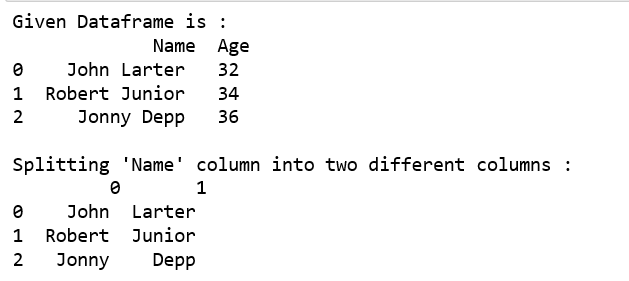Split Name column into “First” and “Last” column respectively and add it to the existing Dataframe .

 `# import Pandas as pd``import` `pandas as pd``  ` `# create a new data frame``df ``=` `pd.DataFrame({``'Name'``: [``'John Larter'``, ``'Robert Junior'``, ``'Jonny Depp'``],``                    ``'Age'``:[``32``, ``34``, ``36``]})``  ` `print``(``"Given Dataframe is :\n"``,df)``  ` `# Adding two new columns to the existing dataframe.``# bydefault splitting is done on the basis of single space.``df[[``'First'``,``'Last'``]] ``=` `df.Name.``str``.split(expand``=``True``)``  ` `print``(``"\n After adding two new columns : \n"``, df)`

Output: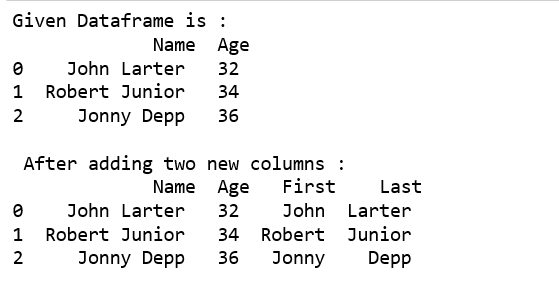Use underscore as delimiter to split the column into two columns.

 `# import Pandas as pd``import` `pandas as pd``  ` `# create a new data frame``df ``=` `pd.DataFrame({``'Name'``: [``'John_Larter'``, ``'Robert_Junior'``, ``'Jonny_Depp'``],``                    ``'Age'``:[``32``, ``34``, ``36``]})``  ` `print``(``"Given Dataframe is :\n"``,df)``  ` `# Adding two new columns to the existing dataframe.``# splitting is done on the basis of underscore.``df[[``'First'``,``'Last'``]] ``=` `df.Name.``str``.split(``"_"``,expand``=``True``)``  ` `print``(``"\n After adding two new columns : \n"``,df)`

Output :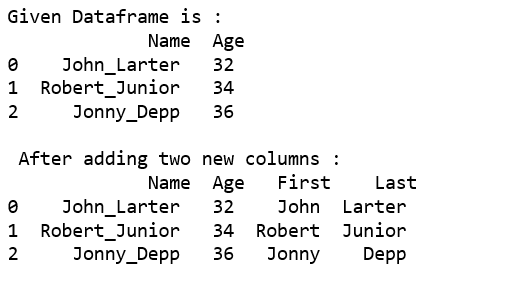Use `str.split()`, `tolist()` function together.

 `# import Pandas as pd``import` `pandas as pd``  ` `# create a new data frame``df ``=` `pd.DataFrame({``'Name'``: [``'John_Larter'``, ``'Robert_Junior'``, ``'Jonny_Depp'``],``                    ``'Age'``:[``32``, ``34``, ``36``]})``  ` `print``(``"Given Dataframe is :\n"``,df)`` ` `print``(``"\nSplitting Name column into two different columns :"``) ``print``(pd.DataFrame(df.Name.``str``.split(``'_'``,``1``).tolist(),``                         ``columns ``=` `[``'first'``,``'Last'``]))`

Output :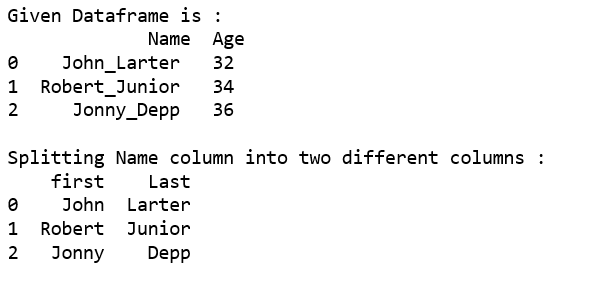Method #2 : Using `apply()` function.

Split Name column into two different columns.

 `# import Pandas as pd``import` `pandas as pd``  ` `# create a new data frame``df ``=` `pd.DataFrame({``'Name'``: [``'John_Larter'``, ``'Robert_Junior'``, ``'Jonny_Depp'``],``                    ``'Age'``:[``32``, ``34``, ``36``]})``  ` `print``(``"Given Dataframe is :\n"``,df)`` ` `print``(``"\nSplitting Name column into two different columns :"``) ``print``(df.Name.``apply``(``lambda` `x: pd.Series(``str``(x).split(``"_"``))))`

Output :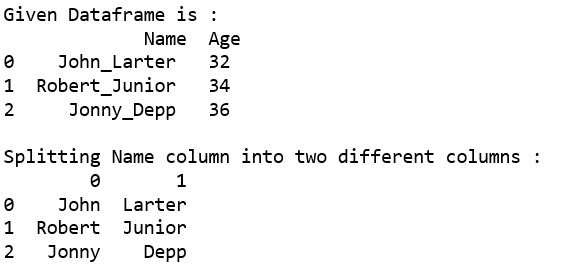Split Name column into two different columns named as “First” and “Last” respectively and then add it to the existing Dataframe.

 `# import Pandas as pd``import` `pandas as pd``  ` `# create a new data frame``df ``=` `pd.DataFrame({``'Name'``: [``'John_Larter'``, ``'Robert_Junior'``, ``'Jonny_Depp'``],``                    ``'Age'``:[``32``, ``34``, ``36``]})``  ` `print``(``"Given Dataframe is :\n"``,df)`` ` `print``(``"\nSplitting Name column into two different columns :"``) `` ` `# splitting 'Name' column into Two columns ``# i.e. 'First' and 'Last'respectively and ``# Adding these columns to the existing dataframe.``df[[``'First'``,``'Last'``]] ``=` `df.Name.``apply``(``   ``lambda` `x: pd.Series(``str``(x).split(``"_"``)))``  ` `print``(df)`

Output :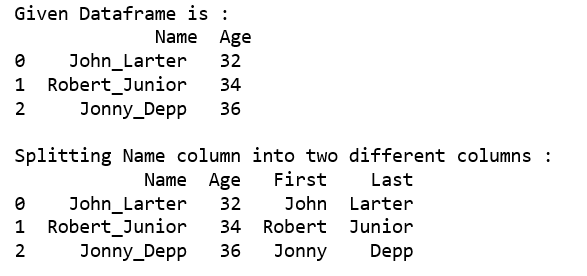Attention geek! Strengthen your foundations with the Python Programming Foundation Course and learn the basics.

To begin with, your interview preparations Enhance your Data Structures concepts with the Python DS Course. And to begin with your Machine Learning Journey, join the Machine Learning – Basic Level Course

My Personal Notes arrow_drop_up